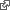#Your NaN is My Zero

I play a little game in my intro to wiki where we sing the 99 Bottles of Beer song. I thought a nice refinement to that page might add bottles back a case at a time.

See Seventh Floor of game.

The song can take a side trip back into the kitchen were cases of beer come in to restock the wall. I wanted to count the cases with the wiki equivalent of an increment.

cases += 1

I was worried about how in my brief detour the case counter would get initialized. No need to worry. It worked.

Cases of Beer 1 SUM Cases of Beer

Hover over the * above will report, can't find value of 'Cases of Beer'. Clear enough for no number.

The third line here uses the SUM markup which says to sum the column of numbers above it. A NaN is like a number that isn't a number and doesn't contribute to the sum. Perfect. When I repeat this on page after page the count worked just as intended.

I don't think this is what the IEEE-754 committee intended when they added NaN, Not a Number, to their definition of floating point arithmetic. wikipediaTheir intention was that NaN would poison any computation with forever more NaN in all subsequent results. If a properly initialized computation went astray, you would know. NaN is the worst. Bad programmer.

# Reduction

The computer language APL introduced the reduce operator / where it and + could be combined to make a summing function +/ which could be applied to vectors.

v = [3 4 5] +/ v ⇒ 12

This was called the plus reduction of v. Notice that APL used ÷ for division so / was free to take on its special role. APL used × for multiplication so ×/ would be the times reduction of a vector.

v = [3 4 5] ×/ v ⇒ 60

This works in wiki too.

3 4 5 PRODUCT Product

I mention APL because one can learn a lot from its attention to detail where it get a lot of details right. For example, the right answer for plus and times reductions of empty vectors might surprise.

v = [ ] +/ v ⇒ 0 ×/ v ⇒ 1

By this computation we can discover the "identity value" under plus and times, 0 and 1 respectively.

Wiki is not so careful as APL when dealing with empty sums and products.

SUM Is the Sum of Nothing.

PRODUCT is the Product of Nothing

Hover over * to see wiki complain, Reduce of empty array with no initial value. From the terminology you can tell it was trying to get this right but falls short. NaN.

# Ruby

Ruby has a variation on reduce called inject which will repeatedly apply a two argument function in a computing loop normally defined by a block for which a symbol will substitute as we do for + and *.

[3,4,5].inject :+ ⇒ 12 [3,4,5].inject :* ⇒ 60

Inject has been carefully coded to not require an initial value when none is given but this is not enough to expose what would be a suitable value for various operations.

[].inject :+ ⇒ nil [].inject :* ⇒ nil

Ruby borrowed the term inject from Smalltalk which borrowed its vector operations from APL where the notion of an identity value associated with plus and times did not transfer well. What is the metaphor here? Inject? What is being injected? The identity value, an initial value, that the programmer provides.

[3, 4, 5].inject {|sum,each| sum + each} ⇒ 12 [3, 4, 5].inject 0, {|sum,each| sum + each} ⇒ 12

Ruby makes the initial value optional by shortening the loop by one iteration when omitted. That is, sum takes on the values 3, 7 in the first case and 0, 3, 7 in the second.

This works for Ruby because the looping is under its control. The computation that started this inquiry involved a user choosing or not choosing to apply a computation repeatedly.

.

See Test Counting With Method where this continues.